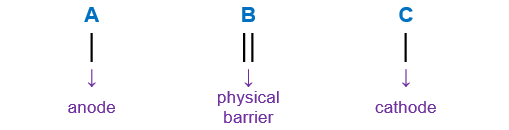# Problem: Consider the following galvanic cell at 25°C:Pt | Cr 2+  (0.30 M), Cr 3+ (2.0 M) | | Co 2+ (0.20 M) | CoThe overall reaction and equilibrium constant value are2Cr 2+(aq) + Co 2+(aq) → 2Cr 3+ (aq) + Co(s)    K = 2.79 X 10 7Calculate the cell potential, ε, for this galvanic cell and ΔG for the cell reaction at these conditions.

###### FREE Expert Solution

Step 1: Identify the anode and the cathode in the reaction and write the overall reaction

Pt | Cr 2+  (0.30 M), Cr 3+ (2.0 M) | | Co 2+ (0.20 M) | Co

When writing a cell notation, we use the following format – “as easy as ABCAnode                                                        Cathode
Pt | Cr 2+  (0.30 M), Cr 3+ (2.0 M) | | Co 2+ (0.20 M) | Co
**Pt is an inert electrode

Cr3+ + e- → Cr2+                          E° = – 0.407 V → anode
Co2+ + 2 e- → Co                         E° =   0.28 V → cathode

lose electrons → oxidation → anode
gain electrons → reduction → cathode

Balance the electrons:

Cathode:     Co2+ + 2 e- → Co
Anode:          [ Cr2+ → Cr3+ + e- ] x 2

Cathode:     Co2+ + 2 e- → Co
Anode:                2 Cr2+ → 2 Cr3+ + 2 e-

Overall reaction: Co2+ + 2 Cr2+ → Co +  2 Cr3+

n = 2 mole e- transferred

Step 2: Calculate the cell potential of the reaction.

Cr3+ + e- → Cr2+                          E° = – 0.407 V → anode
Co2+ + 2 e- → Co                         E° =   0.28 V → cathode

cell = 0.127 V

Step 3: Calculate Ecell using the Nernst Equation.###### Problem Details

Consider the following galvanic cell at 25°C:

Pt | Cr 2+  (0.30 M), Cr 3+ (2.0 M) | | Co 2+ (0.20 M) | Co

The overall reaction and equilibrium constant value are

2Cr 2+(aq) + Co 2+(aq) → 2Cr 3+ (aq) + Co(s)    K = 2.79 X 10 7

Calculate the cell potential, ε, for this galvanic cell and ΔG for the cell reaction at these conditions.